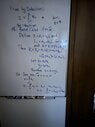# Prove by induction the sum of complex numbers is complex number

• cbarker1

#### cbarker1

Gold Member
MHB
Homework Statement
Prove by induction that for ##z## is a complex then ##\sum_{i=1}^{n}z_i##
Relevant Equations
##P(0)## is true.
If ##P(k)## is true, then ##P(k+1)## is true
See the work below:

I feel like it that I did it correctly. I feel like I skip a step in my induction. Please point any errors.

#### Attachments

•Induction Help.jpg
28.2 KB · Views: 41
Homework Statement:: Prove by induction that for #z# is a complex then #\sum_{i=1}^{n}z_i#
Relevant Equations:: P(0) is true.
If P(k) is true, then P(k+1) is true

See the work below:

I feel like it that I did it correctly. I feel like I skip a step in my induction. Please point any errors.
Hint: Enclose your code in ##, not #.

The steps in your induction are correct. I must ask, though: You know that if y = 0 that z is still a complex number, right?

-Dan

•Greg Bernhardt and cbarker1
Hint: Enclose your code in ##, not #.

The steps in your induction are correct. I must ask, though: You know that if y = 0 that z is still a complex number, right?

-Dan
yes. I do.

The steps are all there, but your notation is bad. You are using ##z## for too many different things. You should distinguish between the sum of n versus the sum of n+1. Call the sum of n ##s_n## and the sum of n+1 ##s_{n+1}##.
Also, it would be a little more clear if, before the last line, you said that ##s_n + z_{n+1}## is the sum of two complex numbers so it is complex.

Last edited:
•cbarker1 and topsquark
In general:

If $$a, b \in S \implies a + b \in S$$ then:$$a_1, \dots a_n \in S \implies \sum_{i=1}^{n}a_i \in S$$

•cbarker1 and topsquark